Use the magnitudes​ (Richter scale) of the earthquakes listed in the data set below. Find the mean and median of this data set. Is the magni

Question

Use the magnitudes​ (Richter scale) of the earthquakes listed in the data set below. Find the mean and median of this data set. Is the magnitude of an earthquake measuring 7.0 on the Richter scale an outlier​ (data value that is very far away from the​ others) when considered in the context of the sample data given in this data​ set? Explain.

in progress 0
5 months 2021-08-19T09:08:16+00:00 1 Answers 21 views 0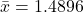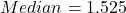7 is an outlier

Step-by-step explanation:

Given

See comment for dataset

Solving (a): The mean

The mean is calculated as: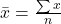So, we have: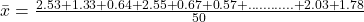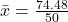Solving (b): The median

First, we sort the data: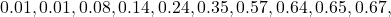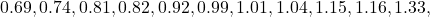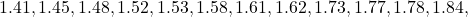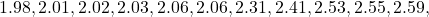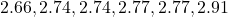The median position is: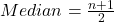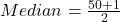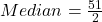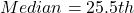This means that the median is the average of the 25th and the 26th item.

So: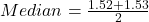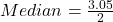Solving (c): Is 7 an outlier

Yes; 7 is an outlier.

Because the range of the dataset (0.01 to 2.92) is far from 7.